# Bars#

## Title: Bars Element#

Dependencies: Matplotlib

Backends: Bokeh, Matplotlib, Plotly

```import numpy as np
import holoviews as hv
hv.extension('matplotlib')
```The `Bars` Element uses bars to show discrete, numerical comparisons across categories. One axis of the chart shows the specific categories being compared and the other axis represents a continuous value.

Bars may also be grouped or stacked by supplying a second key dimension representing sub-categories. Therefore the `Bars` Element expects a tabular data format with one or two key dimensions (`kdims`) and one or more value dimensions (`vdims`). See the Tabular Datasets user guide for supported data formats, which include arrays, pandas dataframes and dictionaries of arrays.

```data = [('one',8),('two', 10), ('three', 16), ('four', 8), ('five', 4), ('six', 1)]

bars = hv.Bars(data, hv.Dimension('Car occupants'), 'Count')

bars
```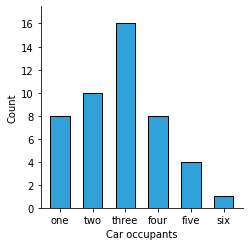A `Bars` element can be sliced and selecting on like any other element:

```bars[['one', 'two', 'three']] + bars[['four', 'five', 'six']]
```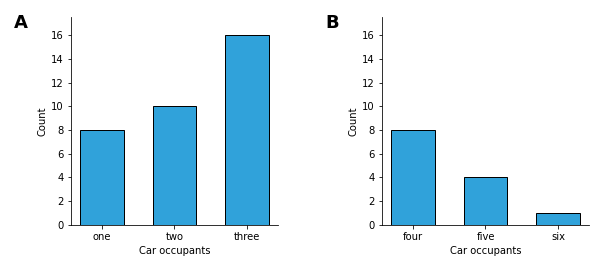It is possible to define an explicit ordering for a set of Bars by explicit declaring `Dimension.values` either in the Dimension constructor or using the `.redim.values()` approach:

```occupants = hv.Dimension('Car occupants', values=['three', 'two', 'four', 'one', 'five', 'six'])

# or using .redim.values(**{'Car Occupants': ['three', 'two', 'four', 'one', 'five', 'six']})

hv.Bars(data, occupants, 'Count')
```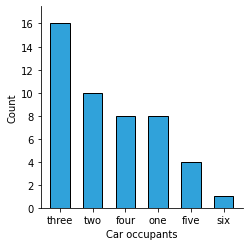`Bars` support nested categorical groupings, e.g. here we will create a random sample of pets sub-divided by male and female:

```samples = 100

pets = ['Cat', 'Dog', 'Hamster', 'Rabbit']
genders = ['Female', 'Male']

pets_sample = np.random.choice(pets, samples)
gender_sample = np.random.choice(genders, samples)

bars = hv.Bars((pets_sample, gender_sample, np.ones(samples)), ['Pets', 'Gender']).aggregate(function=np.sum)

bars.opts(fig_size=300, aspect=2)
```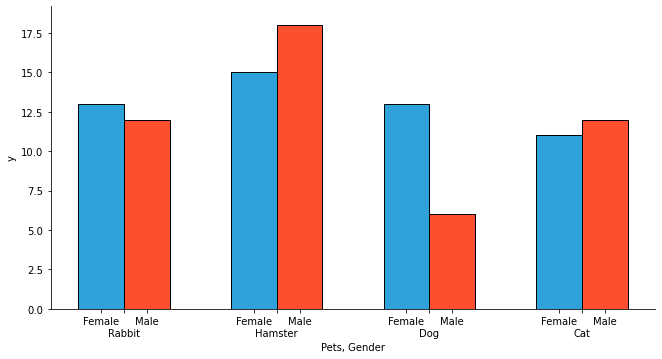Just as before we can provide an explicit ordering by declaring the `Dimension.values`. Alternatively we can also make use of the `.sort` method, internally `Bars` will use topological sorting to ensure consistent ordering.

```(bars.redim.values(Pets=pets, Gender=genders) + bars.sort()).opts(fig_size=300)
```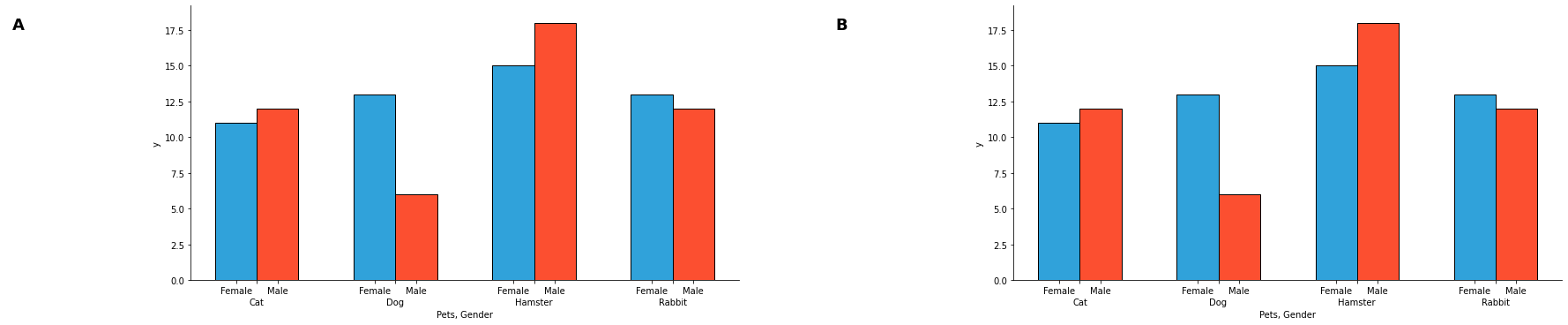To drop the second level of tick labels we can set `multi_level=False`, which will indicate the groupings using a legend instead:

```(bars.sort() + bars.clone().opts(multi_level=False)).opts(fig_size=300)
```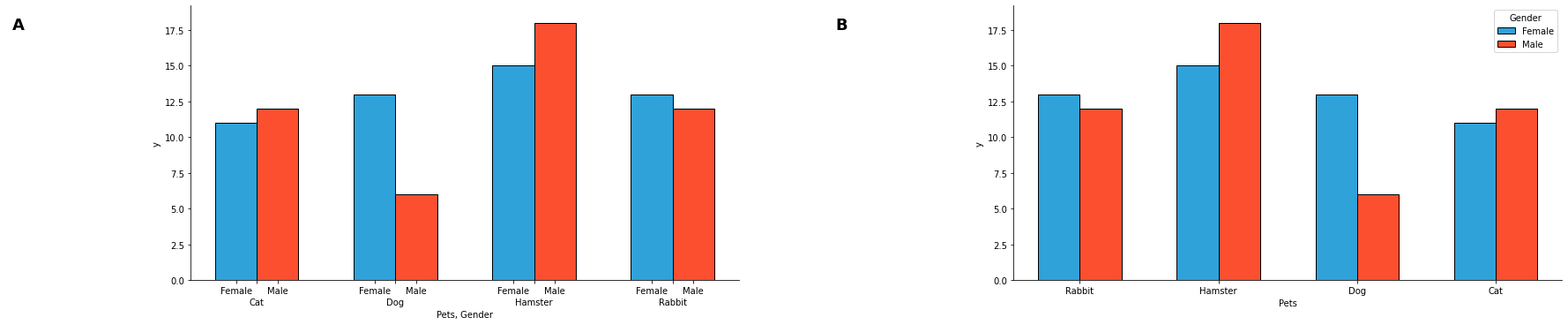Lastly, Bars can be also be stacked by setting `stacked=True`:

```bars.opts(stacked=True)
```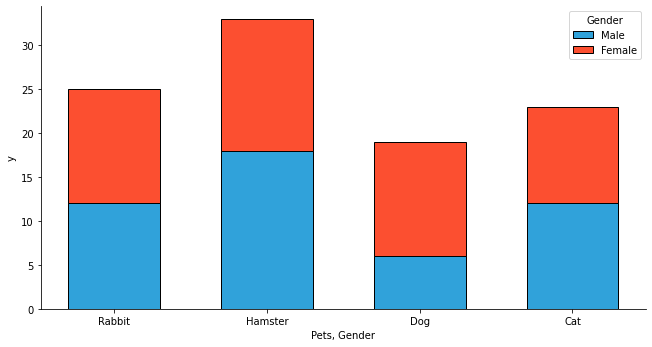For full documentation and the available style and plot options, use `hv.help(hv.Bars).`

This web page was generated from a Jupyter notebook and not all interactivity will work on this website. Right click to download and run locally for full Python-backed interactivity.